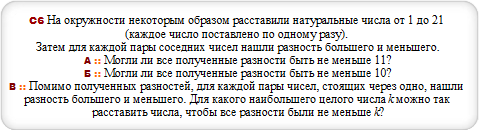# Закажи себе WolframAlpha|Pro! Получи пошаговые решения твоих задач!

## Досрочный ЕГЭ :: 08.05.2014 :: Вариант 5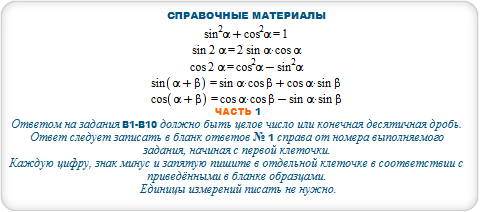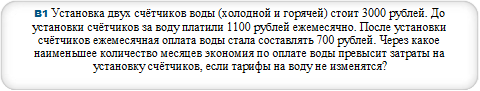#### ceil(a/(b-c)) for a = 3000, b = 1100, c=700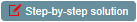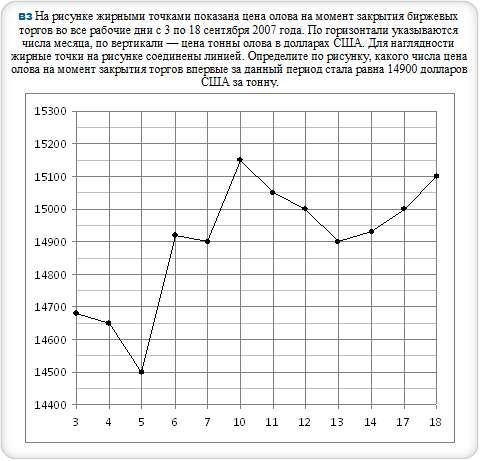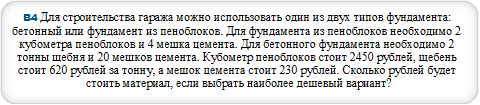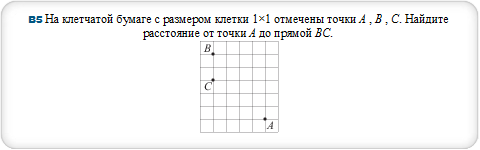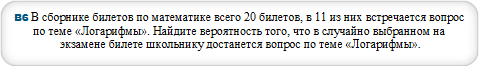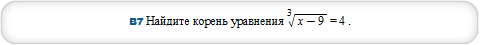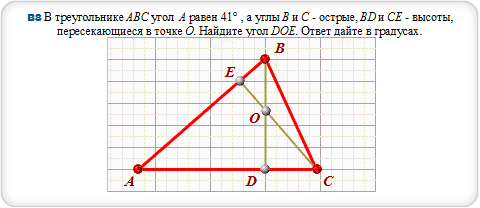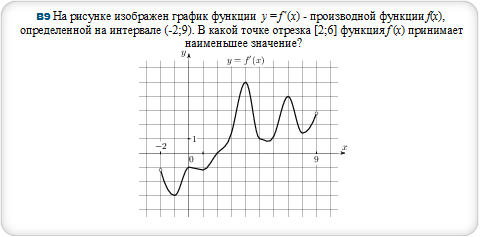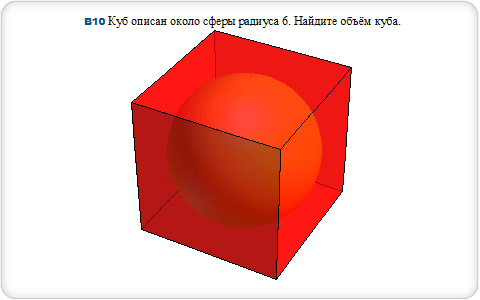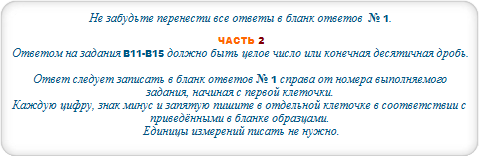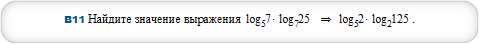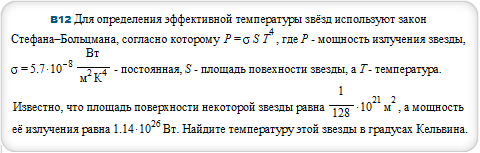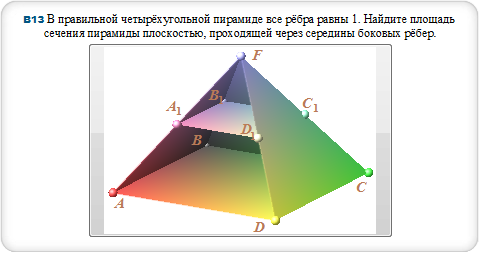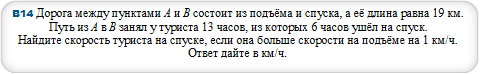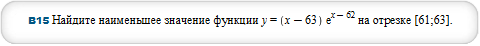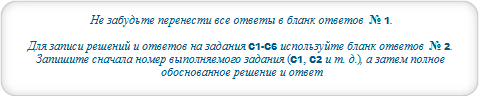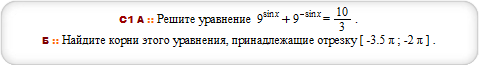#### solve {9^sin(x)+9^(-sin(x)) = 10/3,-7*Pi*(1/2)<=x,x<=-2*Pi}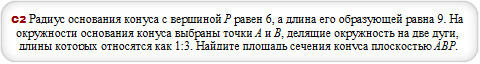#### cone with radius 6, height 3sqrt5 and triangle (-6,0,0),(0,-6,0),(0,0,3sqrt5)#### solve {3^x+54/3^x>=29,log(x+3,(x+1)/4)<=0}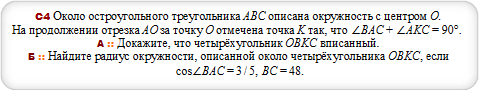#### polygon [7.1, -29.1],[-30, 0],[26, 15],[7.1, -29.1],[46, 0], [26, 15] and circle through [7.1, -29.1],[-30, 0],[26, 15]#### sqrt(x^4+(a-5)^4) = abs(x+a-5)+abs(x-a+5)